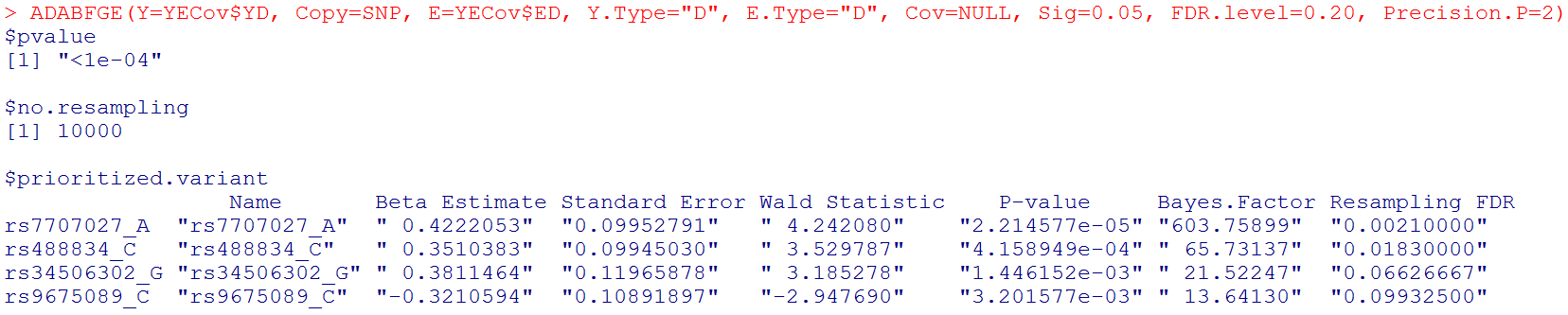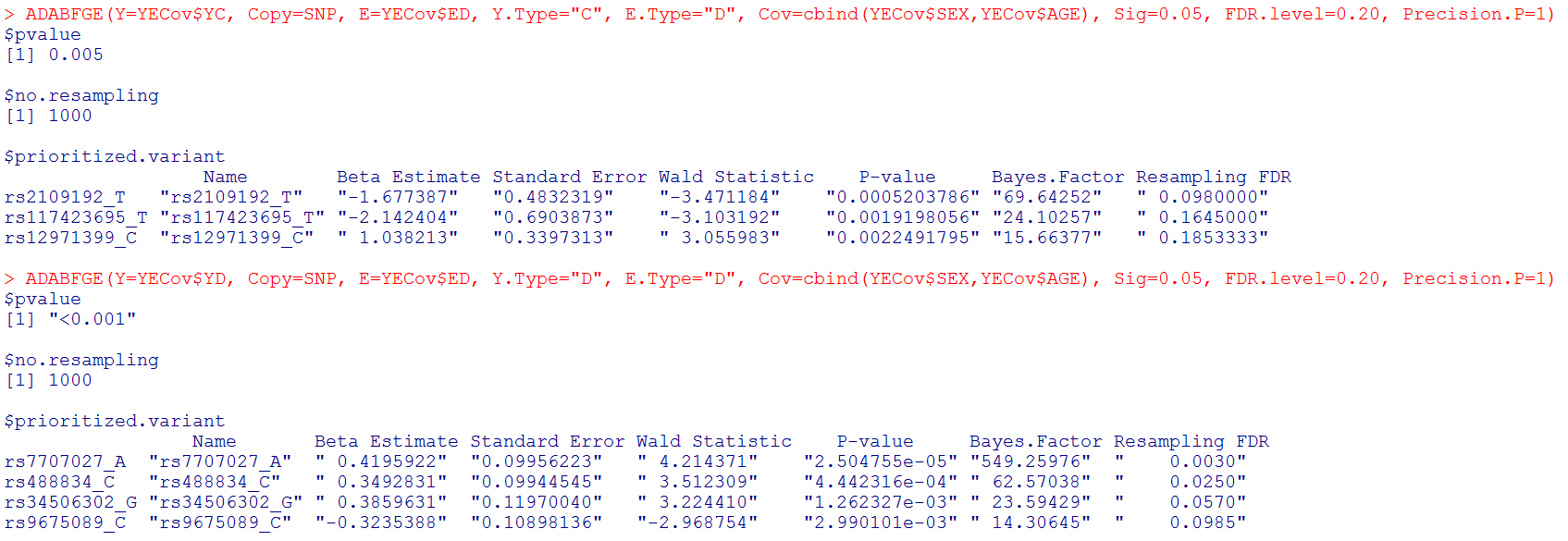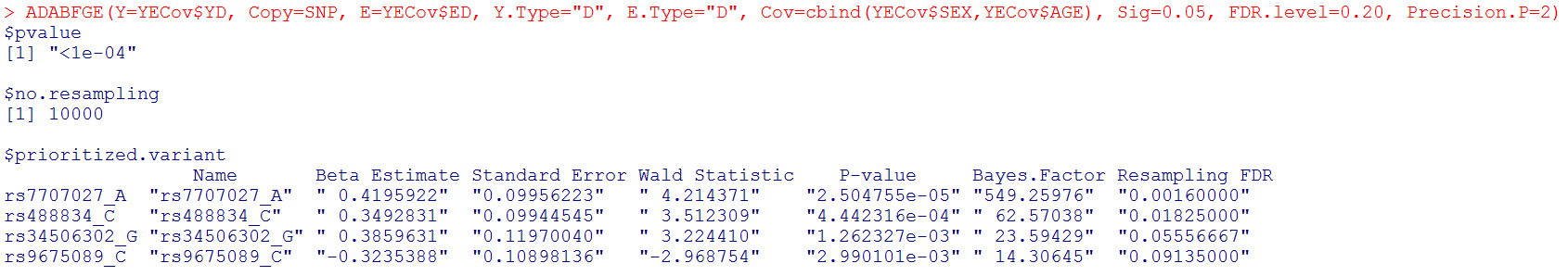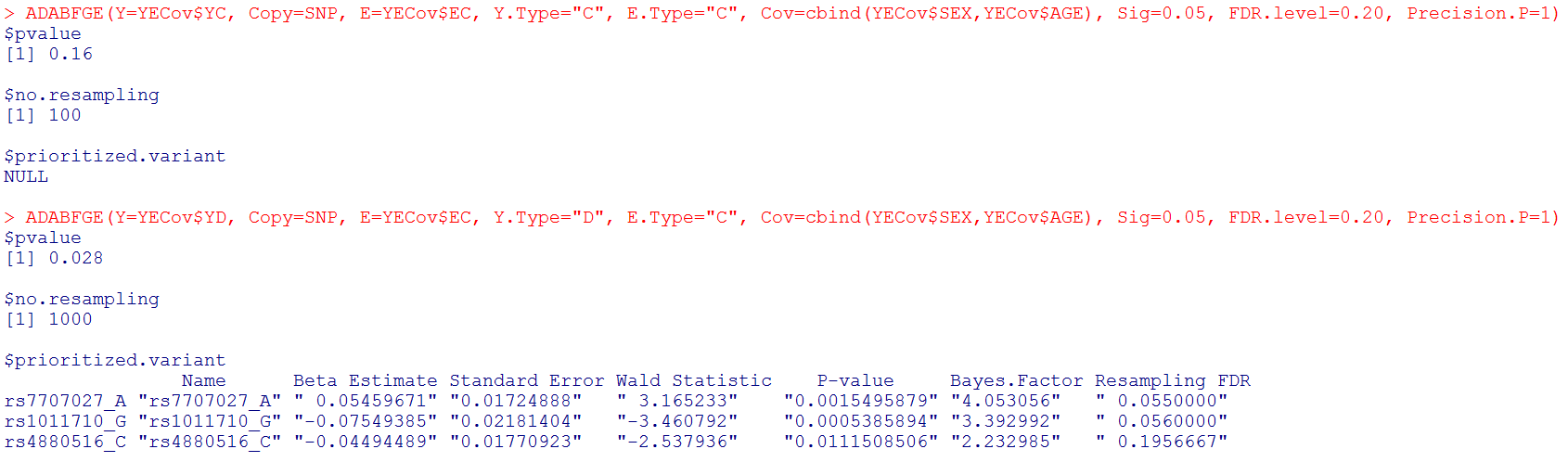Adaptive Combination of Bayes Factors (ADABF) Method as a Powerful Polygenic Test for Gene-Environment Interactions

##########################################################################################
# If you use this code to analyze data, please cite the following paper:
# Lin W-Y*, Huang C-C, Liu Y-L, Tsai S-J, Kuo P-H (2018). Polygenic approaches to detect gene-environment interactions when external information is unavailable. Briefings in Bioinformatics, in press.
# Any questions or comments, please contact: Wan-Yu Lin, linwy@ntu.edu.tw, Institute of Epidemiology and Preventive Medicine, National Taiwan University College of Public Health
# Thank you.
##########################################################################################

The R code to implement the ADABF Polygenic GxE method (file name: "ADABFGEPoly.R")
Toy example:
Phenotype, environmental factor, and Covariates (file name: "YECov.txt")
Genotype (file name: "SNP.txt"), coded as allele counts (0, 1, 2)

Note that the row ordering of subjects must be consistent among the Phenotype file and Genotype file. Missing values are represented by the symbol "NA".

To implement the ADABF method, three R packages, "gtx", "MASS", and "corpcor" need to be installed first. Please use the following R command:

install.packages(c("gtx", "MASS", "corpcor"))Please save our "ADABFGEPoly.R", "YECov.txt", and "SNP.txt" files in a directory, and specify it as the working directory.
In R, the code to implement this function is:

ADABFGE(Y=YECov\$YC, Copy=SNP, E=YECov\$ED, Y.Type="C", E.Type="D", Cov=NULL, Sig=0.05, FDR.level=0.20, Precision.P=1)
ADABFGE(Y=YECov\$YD, Copy=SNP, E=YECov\$ED, Y.Type="D", E.Type="D", Cov=NULL, Sig=0.05,
FDR.level=0.20, Precision.P=1)
ADABFGE(Y=YECov\$YD, Copy=SNP, E=YECov\$ED, Y.Type="D", E.Type="D", Cov=NULL, Sig=0.05,
FDR.level=0.20, Precision.P=2)
ADABFGE(Y=YECov\$YC, Copy=SNP, E=YECov\$EC, Y.Type="C", E.Type="C", Cov=NULL, Sig=0.05,
FDR.level=0.20, Precision.P=1)
ADABFGE(Y=YECov\$YD, Copy=SNP, E=YECov\$EC, Y.Type="D", E.Type="C", Cov=NULL, Sig=0.05,
FDR.level=0.20, Precision.P=1)

ADABFGE(Y=YECov\$YC, Copy=SNP, E=YECov\$ED, Y.Type="C", E.Type="D", Cov=cbind(YECov\$SEX,YECov\$AGE), Sig=0.05,
FDR.level=0.20, Precision.P=1)
ADABFGE(Y=YECov\$YD, Copy=SNP, E=YECov\$ED, Y.Type="D", E.Type="D", Cov=cbind(YECov\$SEX,YECov\$AGE), Sig=0.05,
FDR.level=0.20, Precision.P=1)
ADABFGE(Y=YECov\$YD, Copy=SNP, E=YECov\$ED, Y.Type="D", E.Type="D", Cov=cbind(YECov\$SEX,YECov\$AGE), Sig=0.05,
FDR.level=0.20, Precision.P=2)
ADABFGE(Y=YECov\$YC, Copy=SNP, E=YECov\$EC, Y.Type="C", E.Type="C", Cov=cbind(YECov\$SEX,YECov\$AGE), Sig=0.05,
FDR.level=0.20, Precision.P=1)
ADABFGE(Y=YECov\$YD, Copy=SNP, E=YECov\$EC, Y.Type="D", E.Type="C", Cov=cbind(YECov\$SEX,YECov\$AGE), Sig=0.05,
FDR.level=0.20, Precision.P=1)

where Y is the vector of phenotypes;
Copy is the matrix of allele counts (0, 1, 2);
E is the environmental factor;
Y.Type="D" if Y is dichotomous, whereas Y.Type="C" if Y is continuous;
E.Type="D" if E is dichotomous, whereas E.Type="C" if E is continuous;
Cov is the matrix of covariates;
Sig is the significance level of the ADABF polygenic test (
the default significance level is 0.05, but it can be changed to other values);
FDR.level is the control level of the resampling FDR
(the default FDR control level is 0.20, but it can be changed to other values);
Precision.P is the desired precision level of the P-value. Three levels of Precision.P can be specified by the user:

(1) Precision.P=1, the resampling procedure is repeated until the P-value is larger than 10/B, where B is the number of resampling. The number of resampling would be between 102 and 103. This is the default setting.
(2) Precision.P=2, the resampling procedure is repeated until the P-value is larger than 100/B, where B is the number of resampling. The number of resampling would be between 103 and 104. It takes a longer time than (1).
(3) Precision.P=3, the resampling procedure is repeated until the P-value is larger than 1000/B, where B is the number of resampling. The number of resampling would be between 104 and 105. It takes even a longer time than (2).

Variability of the ADABF P-values: (1) > (2) > (3);
Precision of the ADABF P-values: (3) > (2) > (1);
Time to spend: (3) > (2) > (1).

Note that the numbers of rows in Copy and Cov are the number of subjects.
To know which SNP x E with resampling FDR < 0.20, we encourage you to provide the SNP names in the header line of Copy.
Missing values are represented by the symbol "NA".

The output:#  Output: the P-value of the ADABF test. If the ADABF test is significant, we then prioritize the variants with resampling FDR < 0.20. If the ADABF test is not significant, no variants would be prioritized. The default significance level of the polygenic ADABF GxE test is set to be 0.05, but it can be changed to other values.

# no.resampling is the number of resampling used to calculate the ADABF P-value.

# prioritized.variant shows the SNP x E with resampling FDR < 0.20. The prioritized SNP x E were sorted by their Bayes factors (BFs) rather than P-values. Likewise, the resampling FDR is calculated by comparing the BF with the BFs from the null hypothesis.

#  Please note that "Beta Estimate" is the minor allele x Environment interaction effect. (Recall that "Copy" is the matrix of allele counts (0, 1, 2), and our R function recodes it to be the matrix of minor allele counts.)

#  With the sequential resampling approach, the P-value of the ADABF test may vary. If you wish to have a more precise P-value, please use "Precision.P=2":#  Please note that "Beta Estimate" is the minor allele x Environment interaction effect. (Recall that "Copy" is the matrix of allele counts (0, 1, 2), and our R function recodes it to be the matrix of minor allele counts.)#  If you want to adjust for covariates:#  With the sequential resampling approach, the P-value of the ADABF test may vary. If you wish to have a more precise P-value, please use "Precision.P=2":